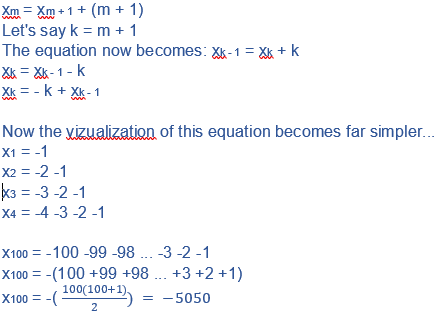# If x1 = -1 and xm = xm+1 - Quantitative Aptitude - CAT Equation

## CAT 2020 - Slot 3 - Quantitative Aptitude - Equation - Question 1 - If x1 = -1 and xm = xm+1

Q. 1: If x(base 1) = -1 and x(base m) = x(base m+1) + (m+1) for every positive integer m, then x(base 100) equals?

1. -5050

2. -5051

3. -5150

4. -5151

Correct Answer: -5050  ( Option 1)### Past Year Question Paper & SolutionsCounselling Session
By IIM Mentor

#### Free Material Area